# Two numbers

Determine the numbers x and y so x + y = 8 is truth and the numbers are in the ratio of 4: 5.

x =  3.5556
y =  4.4444

### Step-by-step explanation:

x+y=8
5x = 4y

x+y=8
5•x = 4•y

x+y = 8
5x-4y = 0

x = 32/9 ≈ 3.555556
y = 40/9 ≈ 4.444444

Our linear equations calculator calculates it.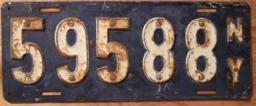Did you find an error or inaccuracy? Feel free to write us. Thank you!Tips to related online calculators
Check out our ratio calculator.
Do you have a linear equation or system of equations and looking for its solution? Or do you have a quadratic equation?

## Related math problems and questions:

• Five numbers in ratioFive integers are in the ratio 1: 2: 3: 4: 5. Their arithmetic mean is 12. Determine the smallest of these numbers.
• Trousers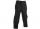Jarek bought new trousers, but the trousers were too long. Their length was in the ratio 5: 8 to Jarek height. Mother his trousers cut by 4 cm, thus the original ratio decreased by 4%. Determine Jarek's high.
• Is right triangleOne angle of the triangle is 36° and the remaining two are in the ratio 3:5. Determine whether triangle is a rectangular triangle.
• Bases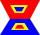The length of the bases trapezium are in ratio 4:5. Length of midline is 15. How long are the bases of a trapezoid?
• Divide 5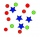Divide 288 in the following ratio 3 : 4 : 5
• Mother and daughter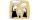The ratio of years mother and daughter is 5:2. After 7 years the ratio is 2: 1. How many years ago daughter was born?
• Number ratio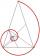Calculate two positive numbers that its ratio is 6:6 and difference was 0.
• Boys and girls 2The ratio of boys to girls in math club is 4:3 . After 8 more girls joined the Club, the ratio become 1:1. How many members are there in the club now?
• Ratio of two unknown numbersTwo numbers are given. Their sum is 30. We calculate one-sixth of a larger number and add to both numbers. So we get new numbers whose ratio is 5:7. Which two numbers were given?
• Translations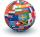If I going to translate the book 6 pages per day I translate it 4 days earlier than if I translated 5 pages a day. If I translate 4 pages a day I translate it for how many days.....?
• Ratio6 numbers are in the ratio 1:5:1:5:5:5. Their sum is 242. What are the numbers?
• Ratio of volumesIf the heights of two cylindrical drums are in the ratio 7:8 and their base radii are in the ratio 4:3. What is the ratio of their volumes?
• Family and age ratiosThe age of father and son are in the ratio 10:3; the age of father and daughter are in the ratio 5:2. How old are father and son if the daughter is 20 years old? In what ratio is age sister and brother?
• Two numbersFind two numbers whose difference and ratio is 2.
• SummandsWe want to split the number 110 into three summands so that the first and the second summand are in the ratio 4: 5, and the third with the first are in ratio 7: 3. Calculate the smallest of the summands.
• Revenue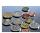Mr. Robert and Mr. Norbert get together € 1400. The revenue split in the ratio of 4:3, depending upon each work. How many got each of them?
• Odd numbersThe sum of four consecutive odd numbers is 1048. Find those numbers ...